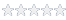# How to repeat a test based on the TestStatus variable

#### Knowledge Base Article # Q200162

Summary How to repeat a test based on the TestStatus variableLogin to rate article
There are a number of ways to repeat a test based on the value of a variable. This example will use the OnEndTest event to repeat test 4.2 up to three times if the test fails. Code in the OnEndTest Event will run at the end of every test, so if the retry is only required on specific tests, then the OnEndEvent code should check for the specific Test Number(s) to prevent retrying all the tests in the application. This example assumes that if Task# 4 Test# 2 fails, then it should be rerun a maximum of 3 times or until it passes. In this example will use a global varaible (m_iTestCount) to keep count of the test retries.  A constant iCount will be used to indicate the maximum number of retries.

Program Global Variables:
! First declare iCount as a Short Constant and give it a value of 3
m_iCount: Short Const = 3 ! Limit the number of retries to 3
m_iTestCount:  Short

Procedure Program.OnEndTest() ! Event
{
if Test.Number = "4.2"   !Check if this is Test Number 4.2

! Check if the test has failed and the number of retries is less than iCount-1
! Remember the test has already been run at least once at this stage
if TestStatus=FAIL AND m_iTestCount < m_iCount-1

! Increment the m_iTestCount variable to track the number of retries
m_iTestCount=m_iTestCount + 1

! Rerun the current test. Remember Index is zero-based
 Login to rate article